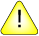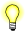This version of this document is no longer maintained. For the latest documentation, see http://www.qnx.com/developers/docs.

# y1(), y1f()

Compute a Bessel function of the second kind

## Synopsis:

```#include <math.h>

double y1( double x );

float y1f( float x );```

## Arguments:

x
The number that you want to compute the Bessel function for.

## Library:

libbessel

Use the -l bessel option to qcc to link against this library.

## Description:

Compute the Bessel function of the second kind for x.

## Returns:

The result of the Bessel function of x.If an error occurs, these functions return 0, but this is also a valid mathematical result. If you want to check for errors, set errno to 0, call the function, and then check errno again. These functions don't change errno if no errors occurred.

## Examples:

```#include <stdlib.h>
#include <stdio.h>
#include <math.h>

int main( void )
{
double x, y, z;

x = j0( 2.4 );
y = y1( 1.58 );
z = jn( 3, 2.4 );

printf( "j0(2.4) = %f, y1(1.58) = %f\n", x, y );
printf( "jn(3,2.4) = %f\n", z );

return EXIT_SUCCESS;
}```

## Classification:

y1() is POSIX 1003.1 XSI; y1f() is Unix

Safety:
Cancellation point No
Interrupt handler No
Signal handler No